# Topological tic-tac-toe

Alex Bolton plays noughts and crosses on unusual surfaces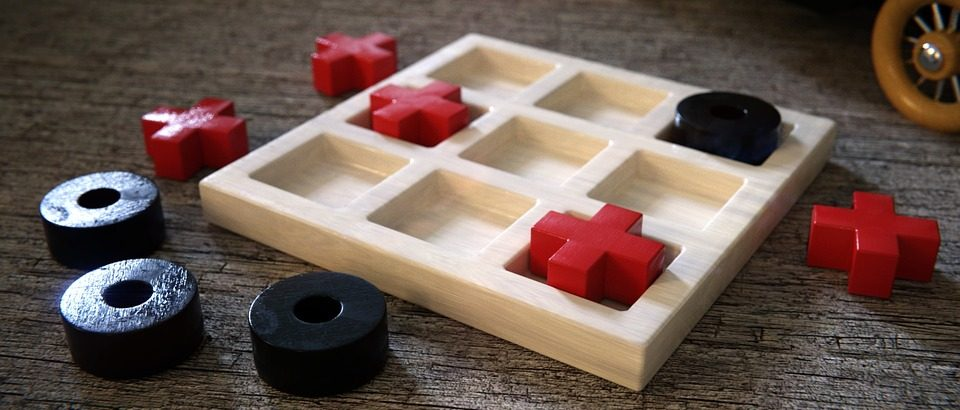Tic-tac-toe also known as noughts and crosses) is a classic game known for its simplicity, and has been popular since ancient times. You and a friend (or enemy!) take turns to mark the squares of a $3 \times 3$ grid. The winner is the first to get three of their symbols in a row (horizontal, vertical or diagonal). A winning game for X is shown below right.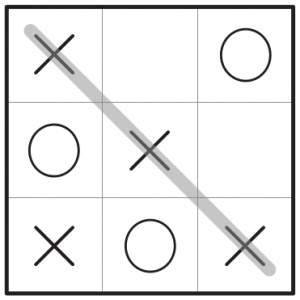A winning game for X

It’s not difficult to work out how to play optimally on a standard board, where you’re guaranteed to at least draw with your opponent. However, what if you’re not restricted to the standard two-dimensional square grid? How would you play then?

To present a fresh challenge and to make tic-tac-toe exciting again, here is a collection of puzzles where you will be swapping your standard square board with one on various topological surfaces. Have fun!

## The cylinder

The first new board to consider is the cylinder. To form a cylinder as in the diagram below, imagine that the board is wrapped around so that the left and right edges of the board are connected to each other, like a piece of paper that has been rolled into a tube. Matching edges are denoted with a $\uparrow$.
In all the subsequent puzzles, it is X’s turn to play, and it is possible for X to win in some number of moves, even if O plays optimally.

#### Puzzle 1: cylinders

How does X win both of these games on cylindrical boards? It is possible to win the first game in one move.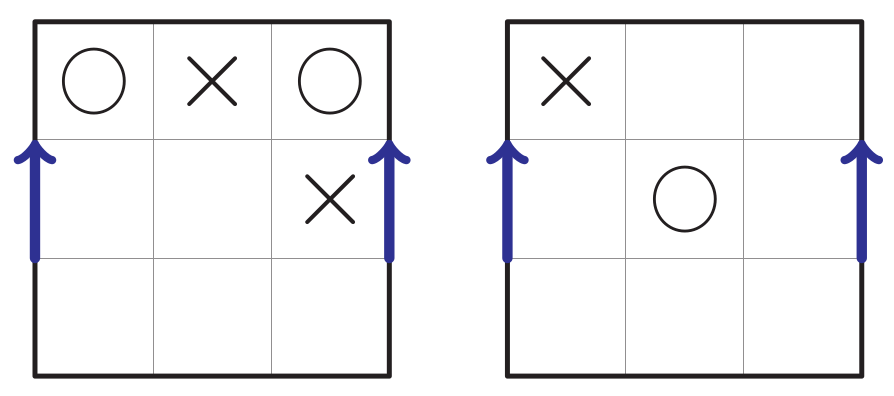The first game can be won in one move. A demonstration of this, along with the folded cylindrical board, is shown below.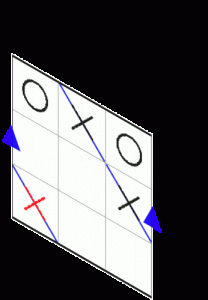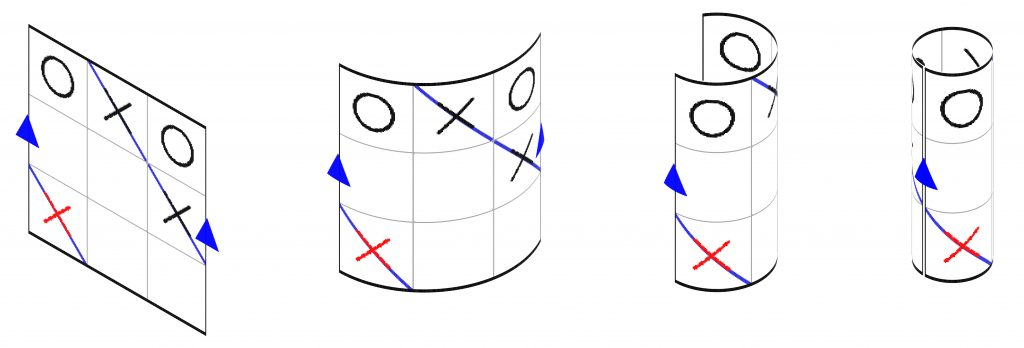Bending the board into a cylinder. The winning move for the first puzzle is shown in red and the winning line is marked in blue.

## The Möbius strip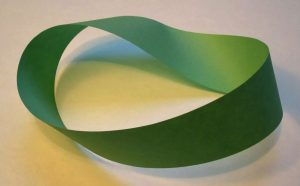A Möbius strip. Image: David Benbennick, CC BY-SA 3.0

How about a Möbius strip? Imagine that the right edge of the board is wrapped around the back of the board and given a half turn, so that it connects to the left edge of the board. The half turn means that top and bottom become flipped as we move off the left or right edge of the board. The edges with a half turn are denoted by a $\uparrow$ and a $\downarrow$. (If you want to actually construct this board, draw each grid square as a wide rectangle so that the grid is wide enough to wrap around with a half twist.)

The figure on below shows adjacent squares in the new board by making copies of the Möbius strip board, and puzzle 2 gives two puzzles on the Möbius strip board.A figure showing which grid squares are adjacent on a Möbius strip board. For example, if you go one place right from the top-right square 3, you will go to the bottom-left square 7.

#### Puzzle 2: Möbius strips

How does X win both of these games on Möbius strip boards? It is possible to win the first game in one move.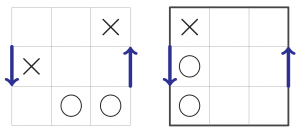## The torus

There’s no need to limit ourselves to only connecting the left and right edges—we can also connect the top and bottom edges. If we return to the cylinder formed by wrapping the right edge of the board round to meet the left edge, we can now connect the top and bottom edges together to form a torus. Now we have two pairs of connected edges, denoted by $\rightarrow$ and $\uparrow$.

For tic-tac-toe purposes, the cylindrical and toroidal boards are identical, and this holds even if we change the game to be `make a line of length $n$ on an $n \times n$ board’ for any $n$. However, for puzzle 3, you need to find a line of length 3 on a $4 \times 4$ board.

#### Puzzle 3: torus puzzle

What should X do to make three in a row on a torus?On the torus, we can think of any row (or column) as being the central one, so it’s easier to prove facts about the torus than for other boards. Have a go at the following challenges.

#### Puzzle 4: more torus puzzles

Can you show that making any line of length $n$ on an $n \times n$ cylindrical board is also a line of length $n$ on an $n \times n$ toroidal board and vice versa (so a game on a torus is equivalent to a game on a cylinder)?

Are any starting positions better than others on a $3 \times 3$ torus?

Is it possible for the game on a $3 \times 3$ toroidal board to end in a draw, with neither player getting 3 in a row (the previous question gives you a shortcut to solving this one)?

## The Klein bottleA figure showing which grid squares are adjacent on Klein bottle board.

Now we will think about playing on a Klein bottle, a shape that cannot be constructed in three dimensions without it intersecting with itself. Fold the top edge of the board over to touch the bottom edge, and connect the left and right edges with a half twist like for the Möbius strip.

The figure to the right shows which squares are adjacent on the Klein bottle board, and puzzle 5 gives two puzzle.

#### Puzzle 5: Klein bottle puzzles

How does X win both of these games on Klein bottle boards? It is possible to win the first game in one move.## The projective plane

Another shape that it is not possible to construct in three dimensions without the shape intersecting itself is the projective plane. This is a shape where the top and bottom edges are connected by a half twist, as are the left and right edges. To imagine how it would be made, think about connecting the single edge of the Möbius strip to itself. (You’ll have to be very dextrous to actually create this from paper!) Puzzle 6 gives two puzzles using the projective plane. Top tip: construct an adjacency map like the one for the Klein bottle.

#### Puzzle 6: projective plane puzzles

How does X win both of these games on projective plane boards?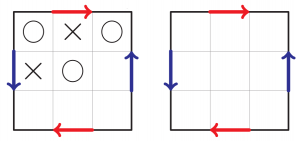(Note: I’m assuming that a valid line comprises three distinct squares, so a single square does not appear more than once in a valid line.)

That brings us to the end of our foray into topological tic-tac-toe. I hope you enjoyed these mind-bending puzzles! The inspiration for this article came from Across The Board by John Watkins, the most complete book on chessboard and other grid puzzles. I would recommend this book for some further interesting puzzles, eg how many queens are needed so that every square on a chessboard is targeted or occupied by one of the queens? The author gives an interesting history of chess problems and shows that they have inspired important advances in maths.